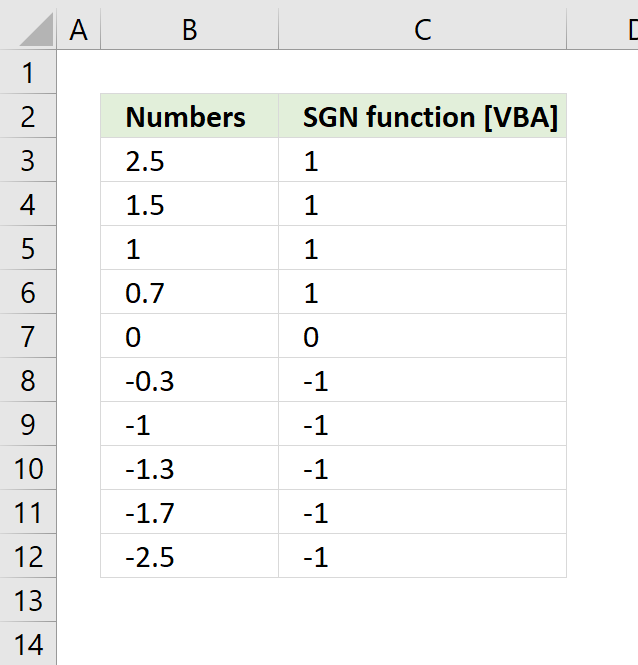Author: Oscar Cronquist Article last updated on August 30, 2018The SGN function returns an integer that shows the sign of the number.

 Argument SGN returns Number greater than 0 (zero). 1 Number equal to 0 (zero). 0 Number less than 0 (zero). -1

SGN(number)

### Arguments

 number Required. Any valid numeric expression. If number is 0 (zero) then 0 (zero) is returned.

```Sub Macro1()